Instant Maths Help:

# Edexcel A Level - Exponential & Log Basics

## Exponential Functions, Logs & Log Laws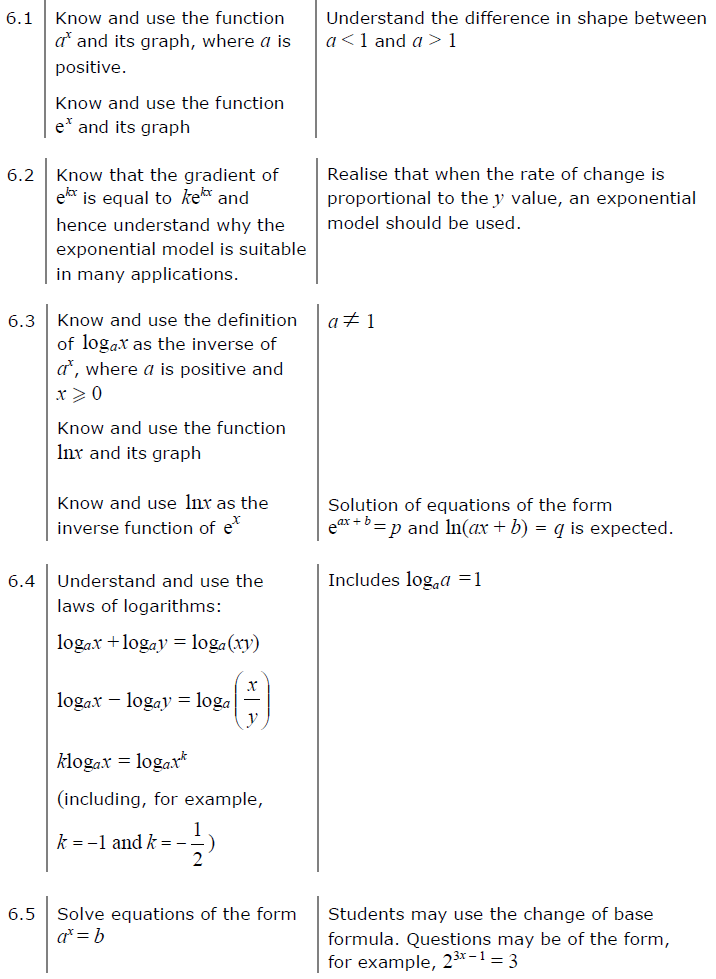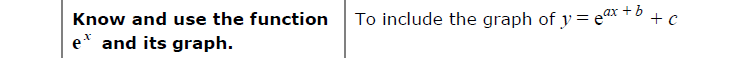##### Edexcel 9MA0/02 Jun 2023 A2 Exam Q. 3 :   5 marks in 6:00 min.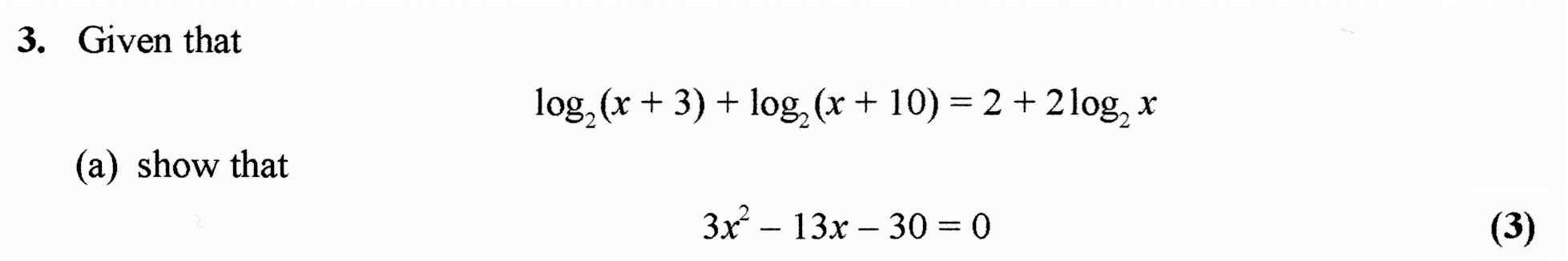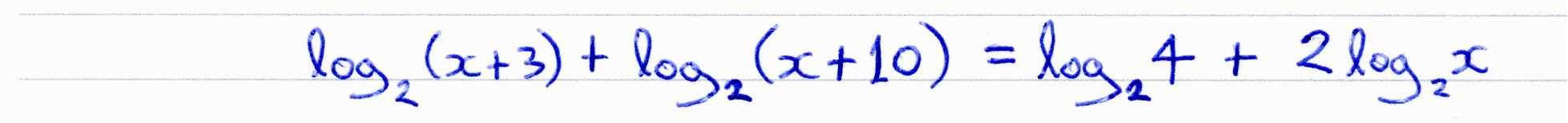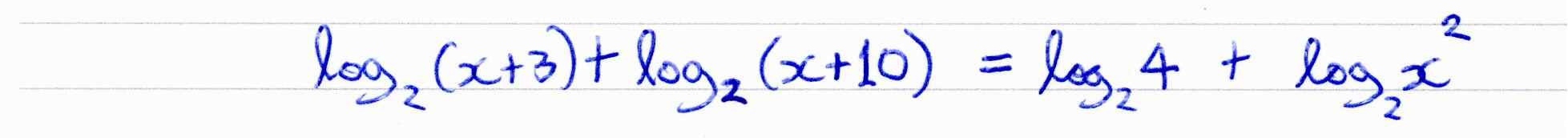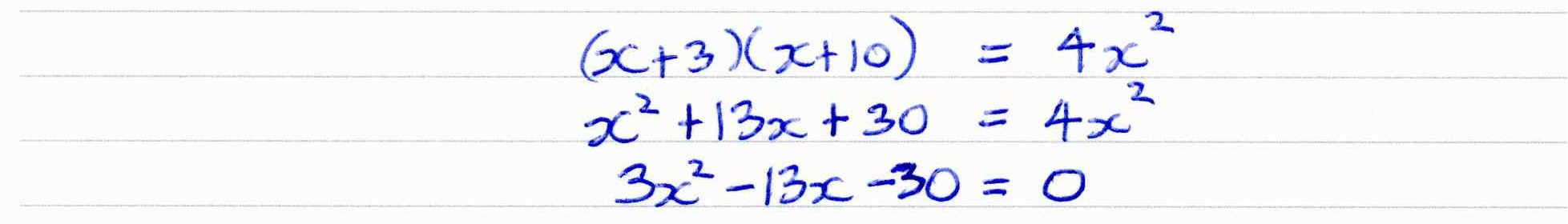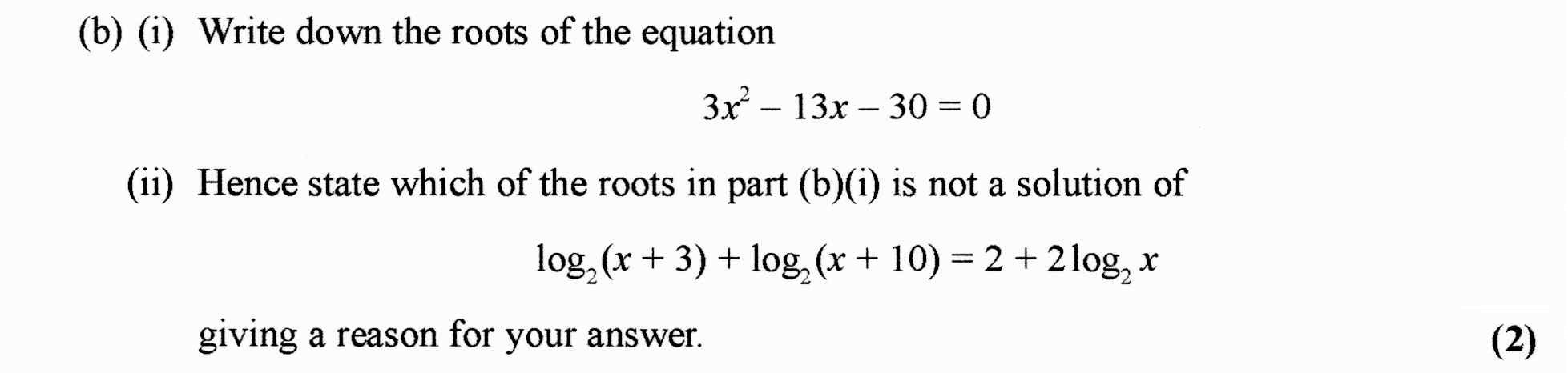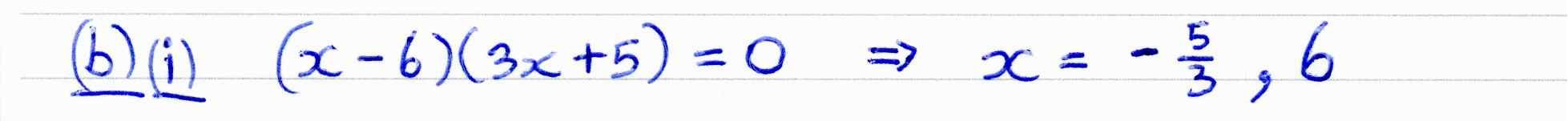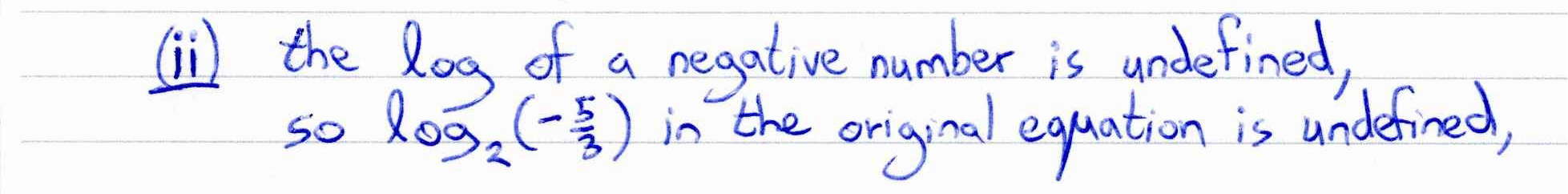##### Edexcel 9MA0/01 Jun 2023 A2 Exam Q. 6 :   6 marks in 7:12 min.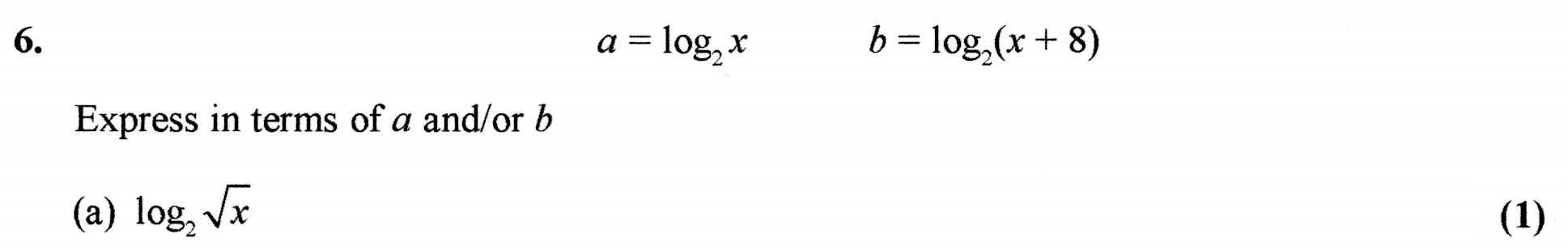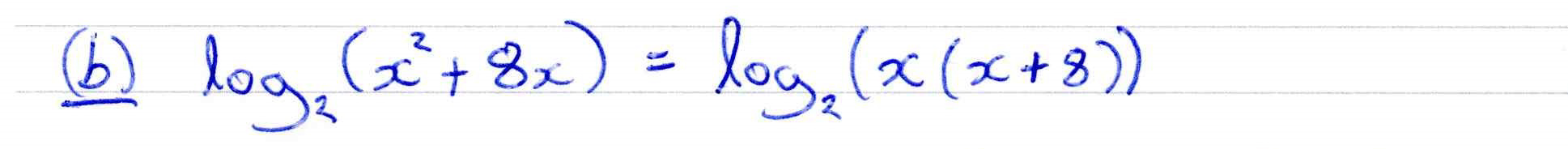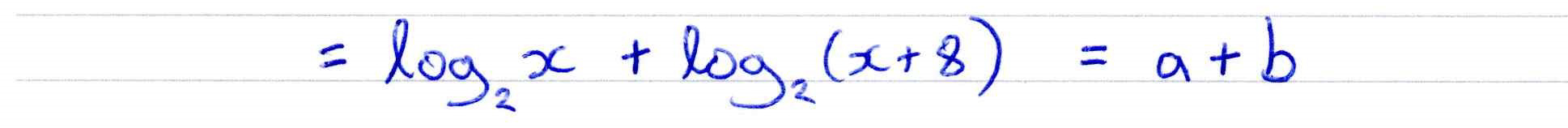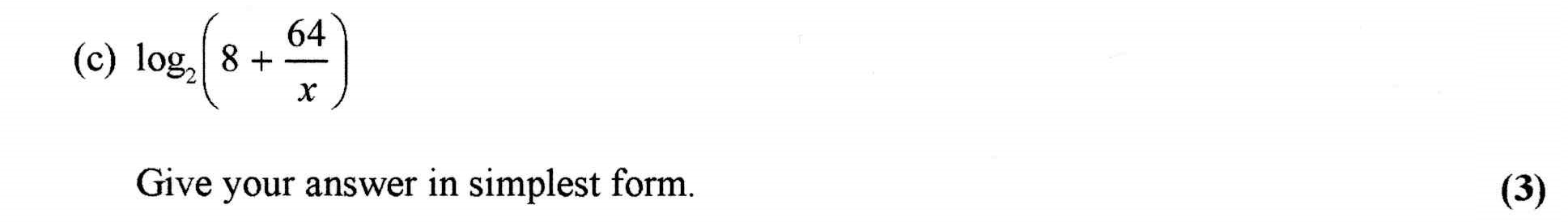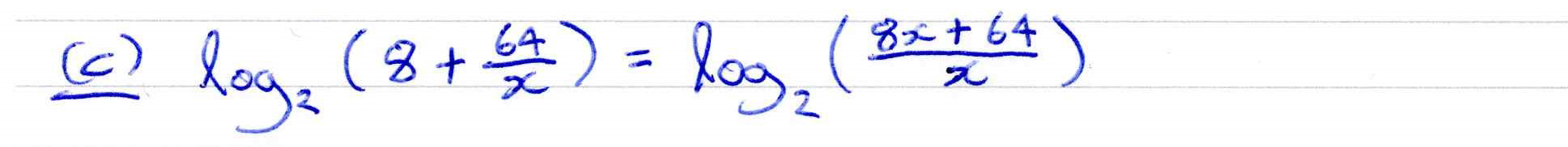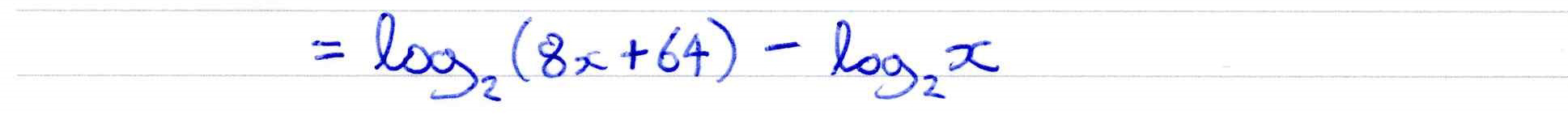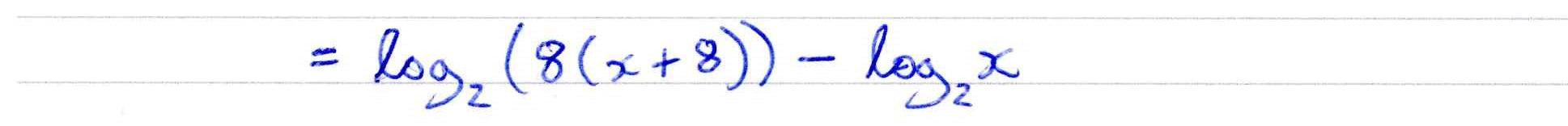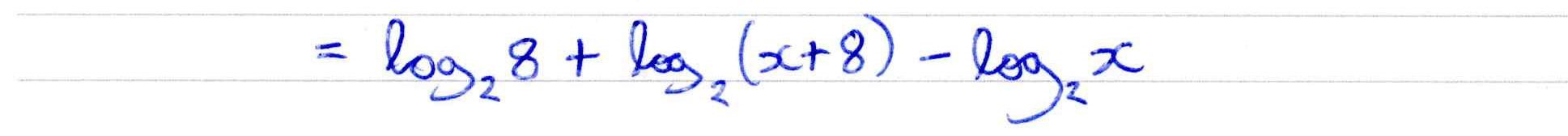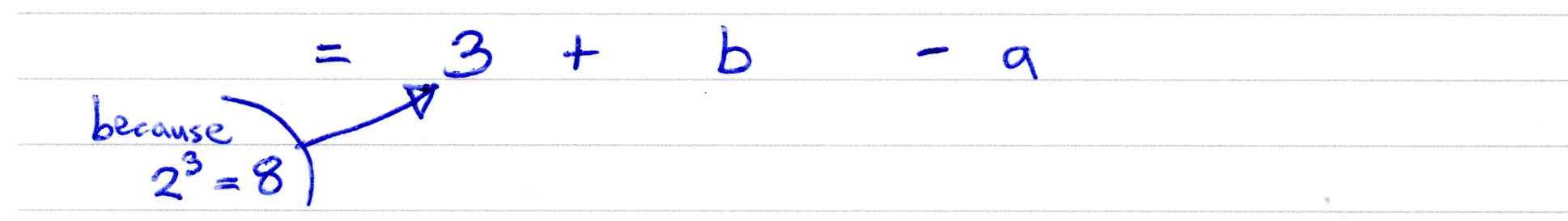##### Edexcel 8MA0/01 Jun 2023 AS Exam Q. 9 :   5 marks in 6:00 min.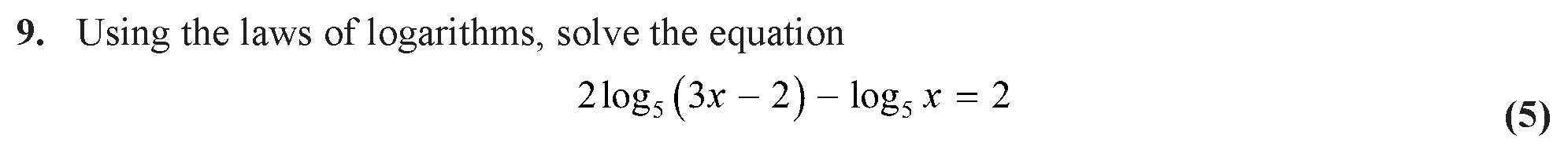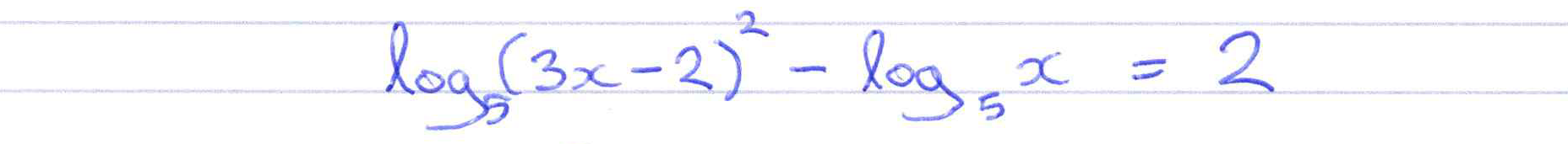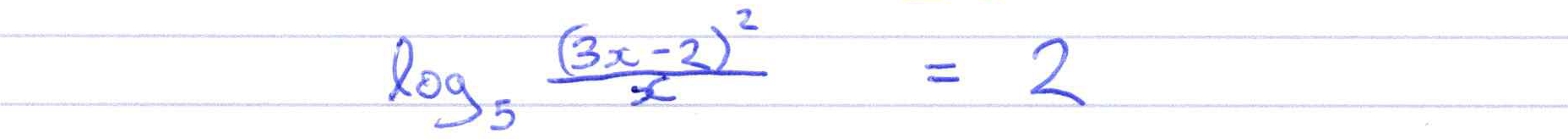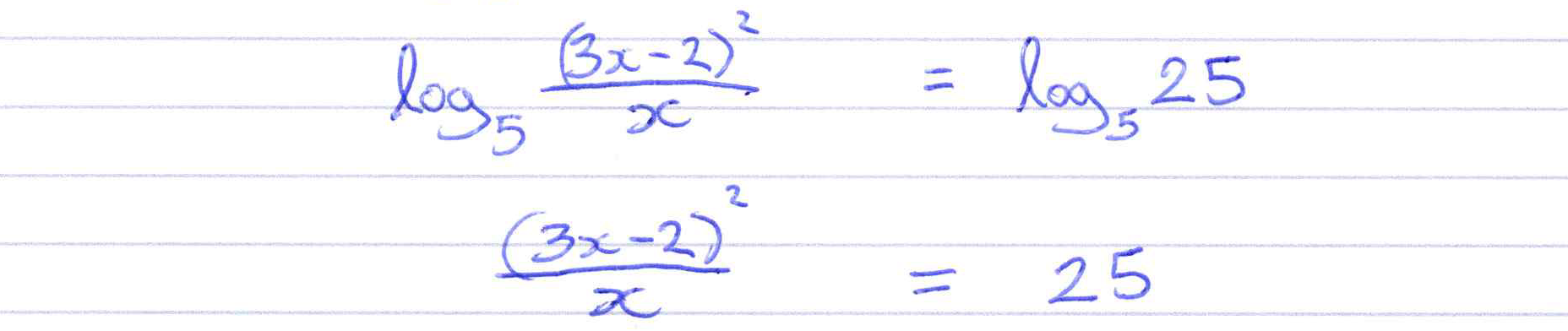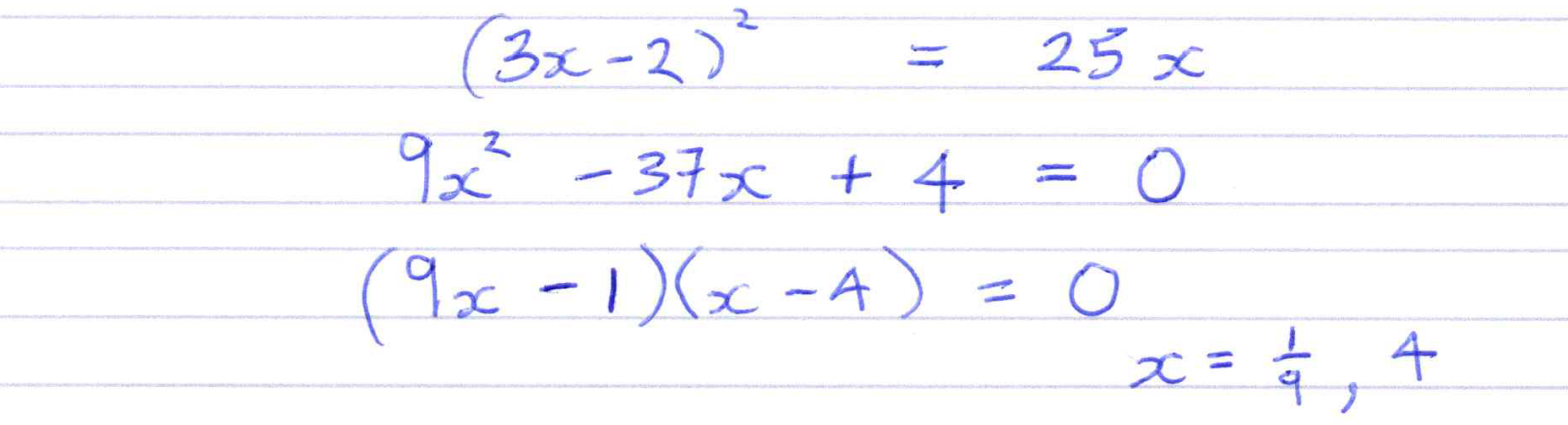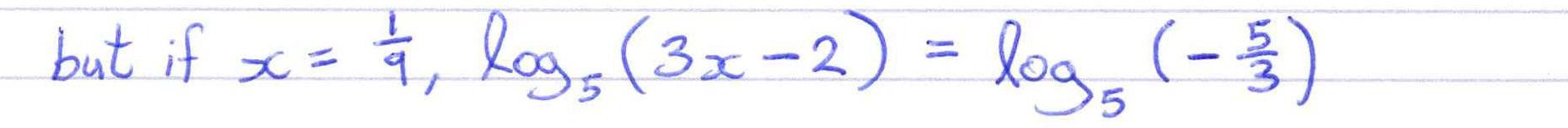##### Using exponential functions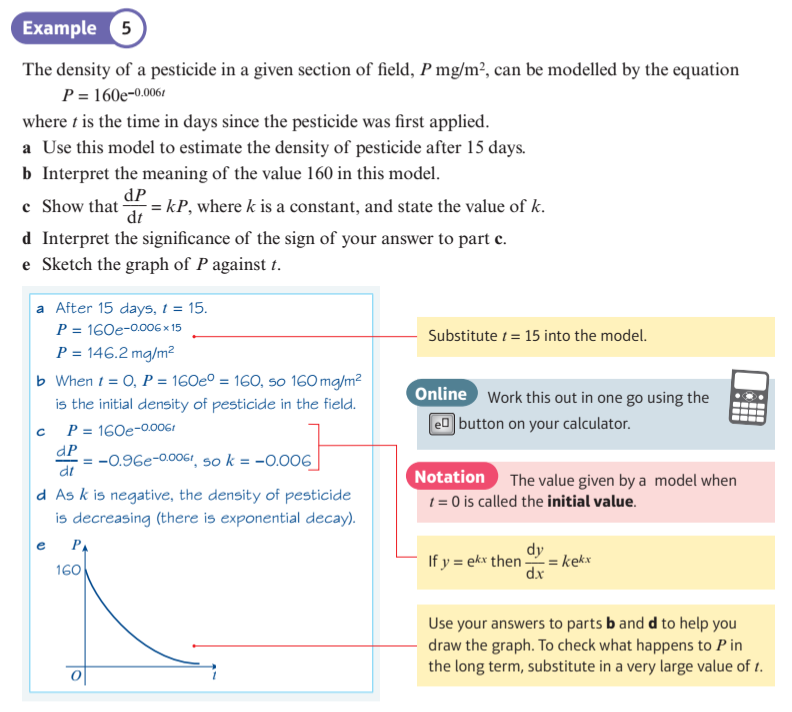##### Using logarithms to any base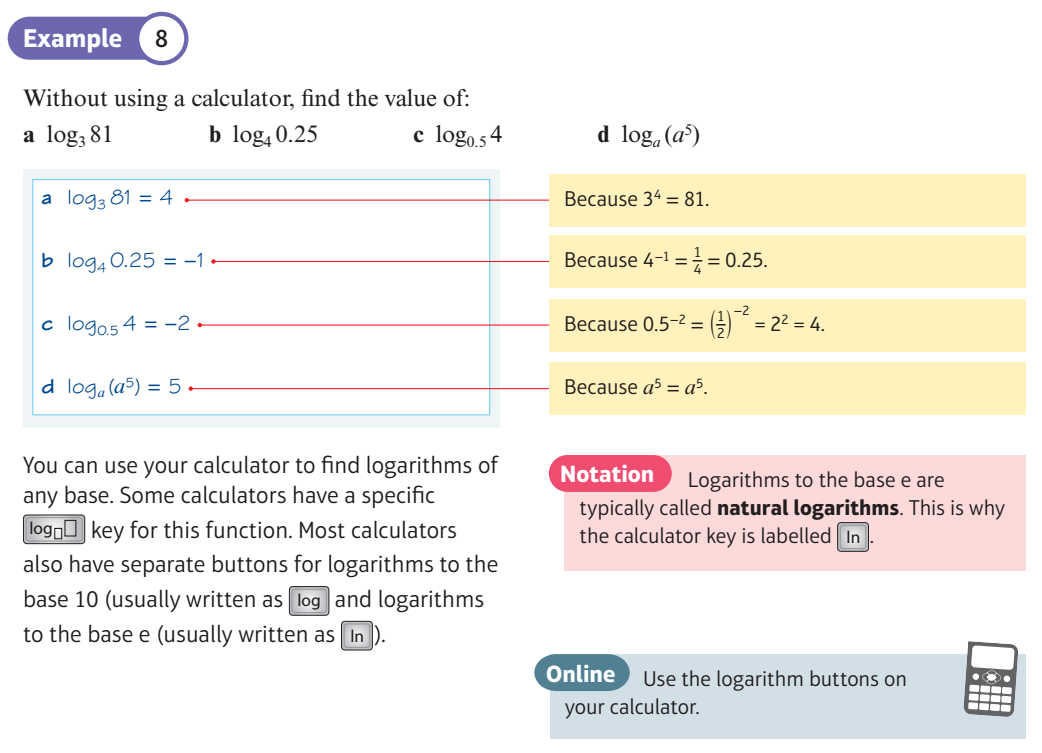##### Exponential Graphs: ${a^x}$
The graph of $\color{blue}{ f(x) = {a^x} }$ is shown in blue, together with its tangent and gradient at a sample point in red.

By considering the gradient at x = 0, 1, 2, 3, can you predict what the gradient function of $\color{blue}{ f(x) = {a^x} }$ is?
##### Gradients of ${a^x}$ and ${e^x}$
Compare the graph of $$\color{blue}{ f(x) = {a^x} }$$ with the graph of its gradient function, $$\color{green}{ f'(x) }$$.
Adjust the value of a till the the two curves coincide.